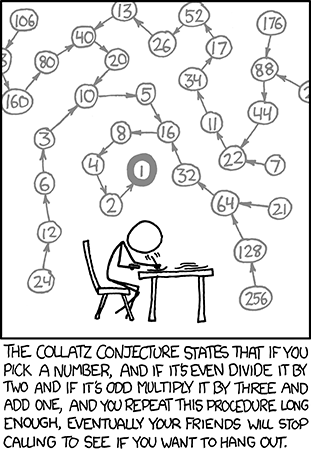In class, we discussed a certain method called collatz. In this brief note, we will discuss the significance and subtlety of the Collatz function.

Consider the following method:

 1 2 3 4 5 6 7 8 9 10 11 12 13 public static long collatz (long n) { if (n == 1) { return 1; } if (n % 2 == 0) { return collatz (n / 2); } else { return collatz (3 * n + 1); } }

So what does collatz(n) actually do? A little less formally, we can describe the behavior as follows:

1. write down n
2. if n is 1, stop. Otherwise…
3. if n is even, divide n by 2, and go back to step 1.
4. otherwise (if n is odd), multiple n by 3, add 1, and go back to step 1.

This process description is not terribly complicated, but its behavior is incredibly subtle.

The first thing that we might ask about a recursively defined function is whether or not it enters an infinite loop. If you apply the process above for several starting values of n, they will probably all end up at 1, and the function will terminate without hitting an infinite loop. In fact, for all numbers n up to about $$10^{21}$$, collatz(n) is known not to hit an infinite loop on input n. But in general it is unknown if there is any positive integer n for which collatz(n) enters an infinite loop! I find this fact truly remarkable, and it is a testament to the subtlety of recursion. We can write a method with 4 lines of code, and no one knows whether or not the method will enter an infinite loop!

The Collatz Conjecture also known as the 3 x + 1 problem posits that the process above stops—i.e., eventually reaches 1—for every positive integer $$n$$. This is a notoriously difficult open problem. Some of most celebrated mathematicians of the last 80 years have worked on the Collatz Conjecture, yet it is still described as “an extraordinarily difficult problem, completely out of reach of present day mathematics” (Lagarias 2010).

Quite recently, Terence Tao proved that the Collatz Conjecture “almost” holds for “almost all” natural numbers $$n$$ (Tao 2019). Yet even with this substantial progress, he claims that “[e]stablishing the conjecture for all $$n$$ remains out of reach of current techniques.” Previously in 2011, Tao stated in a blog post that

[o]pen questions with this level of notoriety can lead to what Richard Lipton calls “mathematical diseases” (and what I termed an unhealthy amount of obsession on a single famous problem). (See also this xkcd comic regarding the Collatz conjecture.) As such, most practicing mathematicians tend to spend the majority of their time on more productive research areas that are only just beyond the range of current techniques. Nevertheless, it can still be diverting to spend a day or two each year on these sorts of questions, before returning to other matters; so I recently had a go at the problem. Needless to say, I didn’t solve the problem, but I have a better appreciation of why the conjecture is (a) plausible, and (b) unlikely be proven by current technology…

Randall Munroe aptly describes many people’s fascination and frustration with the Collatz Conjecture with the following webcomic (source):